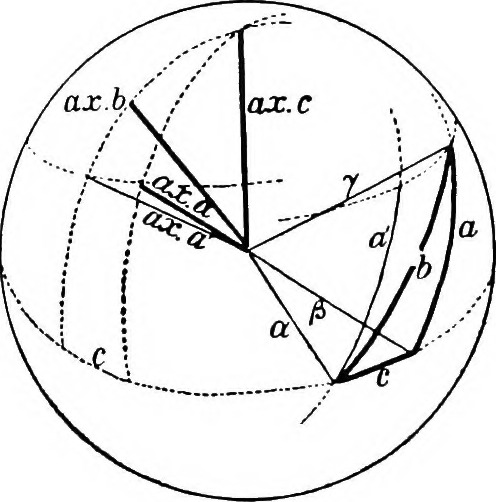maths

## Geometry of division rings

It is fairly easy to construct a geometry from algebra: given a division ring K we form an n-dimensional vector space, the points being the elements of the field and a line being a translation of all (left) multiples of a non-zero vector, i.e. of the form $$\{a\mathbf{v} + \mathbf{c}| a \in K\}$$ for some fixed vectors $$\mathbf{v} \neq 0$$ and c.

Interestingly it’s just as possible to go the other way, if we’re careful about what we mean by a geometry. I will loosely follow Artin’s book Geometric Algebra. In particular we have the undefined terms of point, line and the undefined relation of lies on. Then, for a fixed positive integer, the axioms are:

1. Given two distinct points there is a unique line that both points lie on
2. Each line has at least three points which lie on it
3. Given a line and a point not on that line there exists a unique line lying on the plane containing them that the point lies on and no point of the first line lies on.
4. All points are spanned by d+1 points and no fewer.